• 要求：输入一个Excel公式，使用Java代码执行此公式！ 代码： package com.wangyk.springboot.utils.utils; import lombok.extern.slf4j.Slf4j; import org.apache.poi.hssf.usermodel.*; import org.apache.poi....

要求：输入一个Excel公式，使用Java代码执行此公式！

代码：

package com.wangyk.springboot.utils.utils;

import lombok.extern.slf4j.Slf4j;
import org.apache.poi.hssf.usermodel.*;
import org.apache.poi.ss.formula.FormulaParseException;
import org.apache.poi.ss.usermodel.CellType;
import org.apache.poi.ss.usermodel.FormulaEvaluator;

/**
* @description:
* @create: 2021-03-11 22:51
**/
@Slf4j
public class ExcelFormulaUtil {

/**
* Sheet 中的每一行
*/
private static HSSFRow row = null;

private static FormulaEvaluator formulaEvaluator = null;

/**
* 初始化一个 HSSFWorkbook
*/
static {
HSSFWorkbook workbook = new HSSFWorkbook();
HSSFSheet sheet = workbook.createSheet();
row = sheet.createRow(0);
formulaEvaluator = new HSSFFormulaEvaluator(workbook);
}

/**
* 计算值
*
* @param formula Excel 中的公式，例如：MAX(56-FLOOR(20/6,1),2)
* @return
*/
public static int caculateFormula(String formula) {
// 这里必须新建一个对象，否则只有第一个 formula 才有效。查看 formulaEvaluator.evaluate 的源码。
HSSFCell cell = row.createCell(0);
cell.setCellType(CellType.FORMULA);
try {
cell.setCellFormula(formula);
return (int) Math.round(formulaEvaluator.evaluate(cell).getNumberValue());
} catch (FormulaParseException e) {
log.error(formula + "parse Exception: ", e);
return 0;
}
}
}

展开全文poi excel java
• 这是我自己写的一个 Java 仿 Excel 公式计算 工具，支持自定义公式 #

这是我自己写的一个 Java 仿 Excel 公式计算 工具，支持自定义公式

多页签Excel公式计算

公式：

'交易性-股票'N3=现金!A1*银行存款!C1+ABS(MIN(O2+2,银行存款!B3:银行存款!E5)*2-MAX('交易性-股票'!A1:'交易性-股票'!D3))+MAX(现金!A2:现金!O3)

Excel计算结果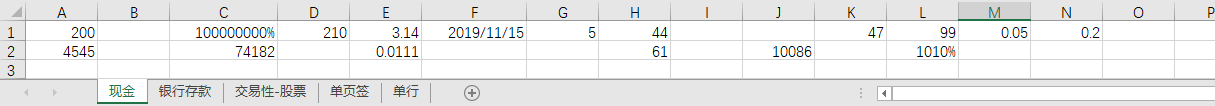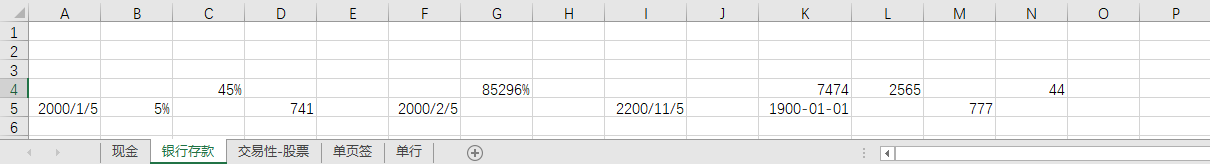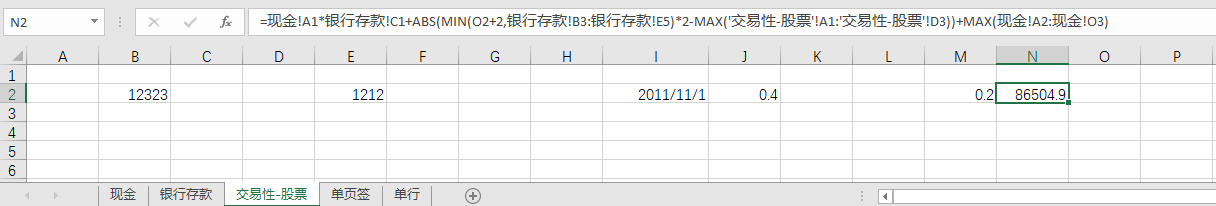Java计算结果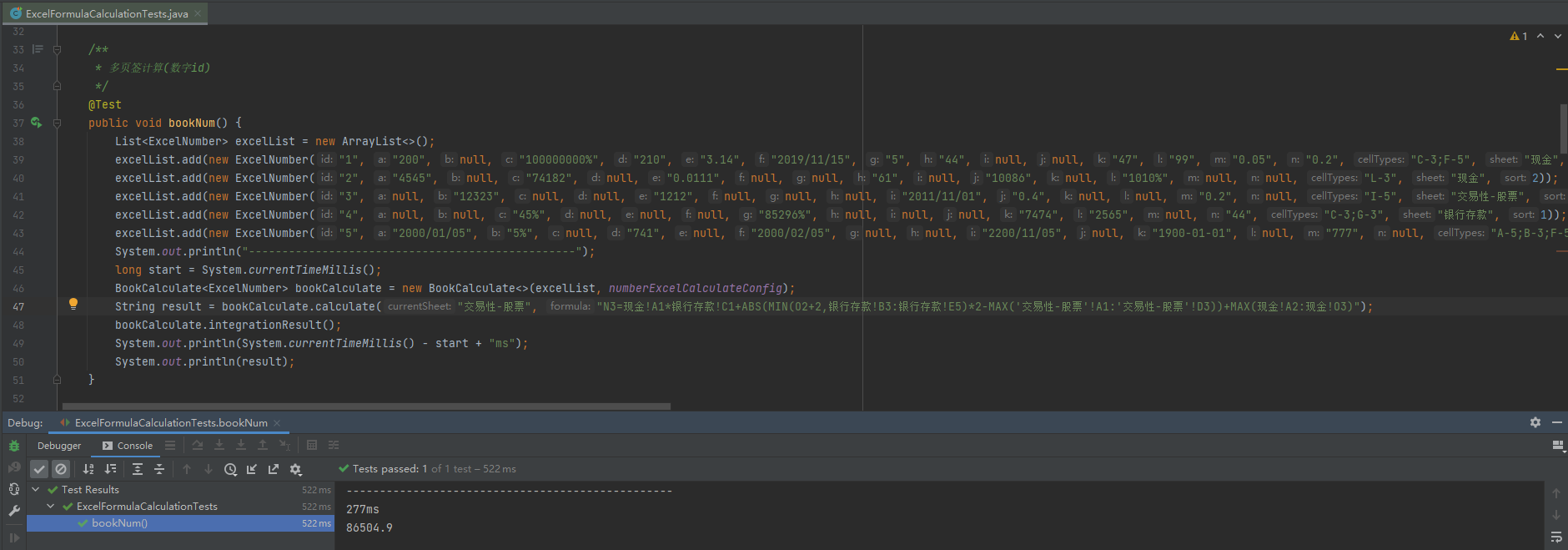单页签Excel公式计算

公式

K5=A1+ABS(MIN(C5+2,A1:I5)*2-MAX(L1:N5))+MAX(A2:J2)

N5=ROUND(2*K5+5,0)

Excel计算结果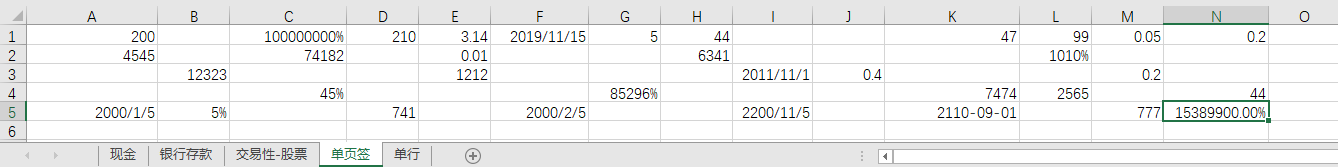Java计算结果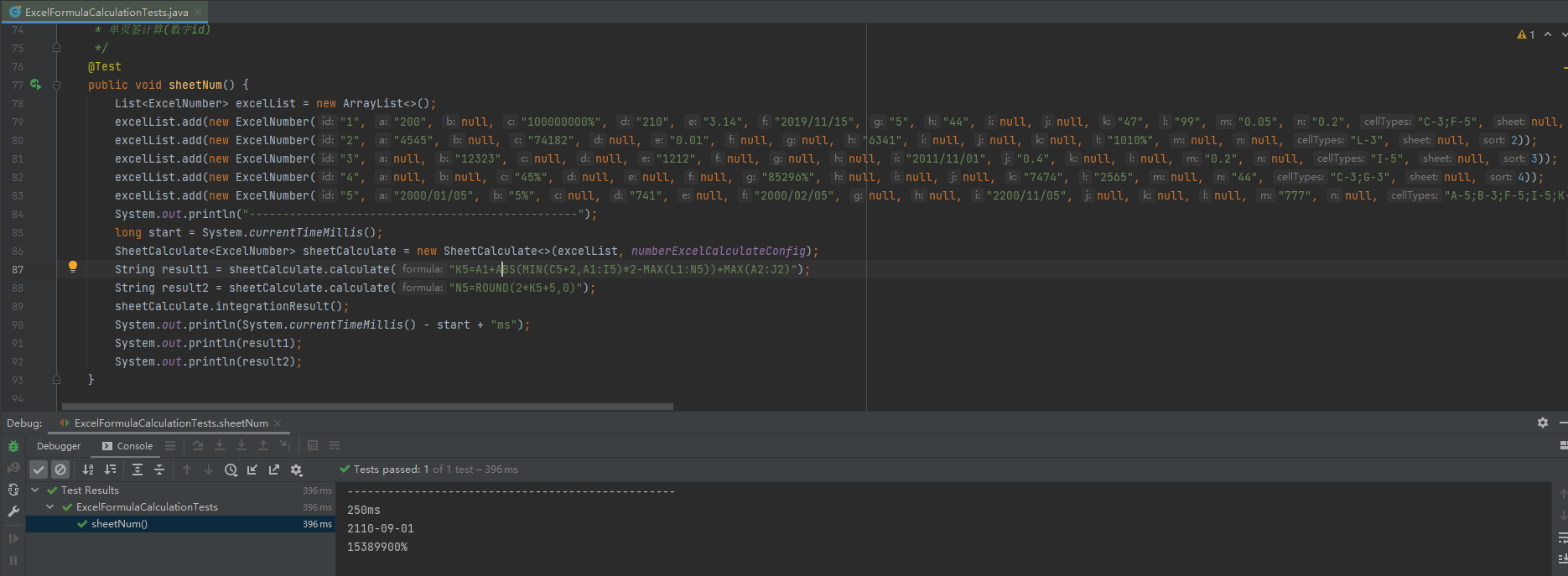单行计算

公式

I=A+ABS(MIN(O+2,B)*2-MAX(A:H))+MAX(K:N)

J=ROUND(2*I+5,0)

Excel计算结果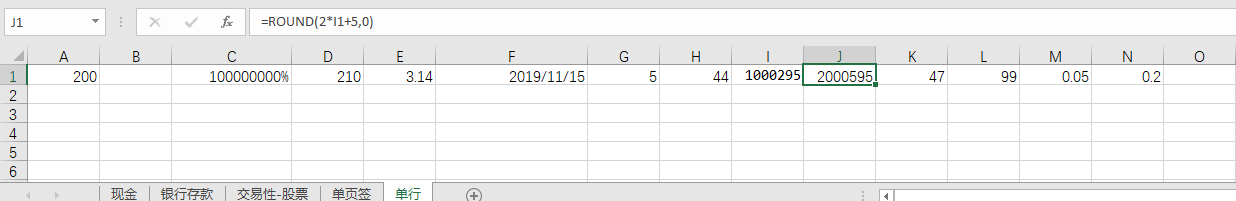Java计算结果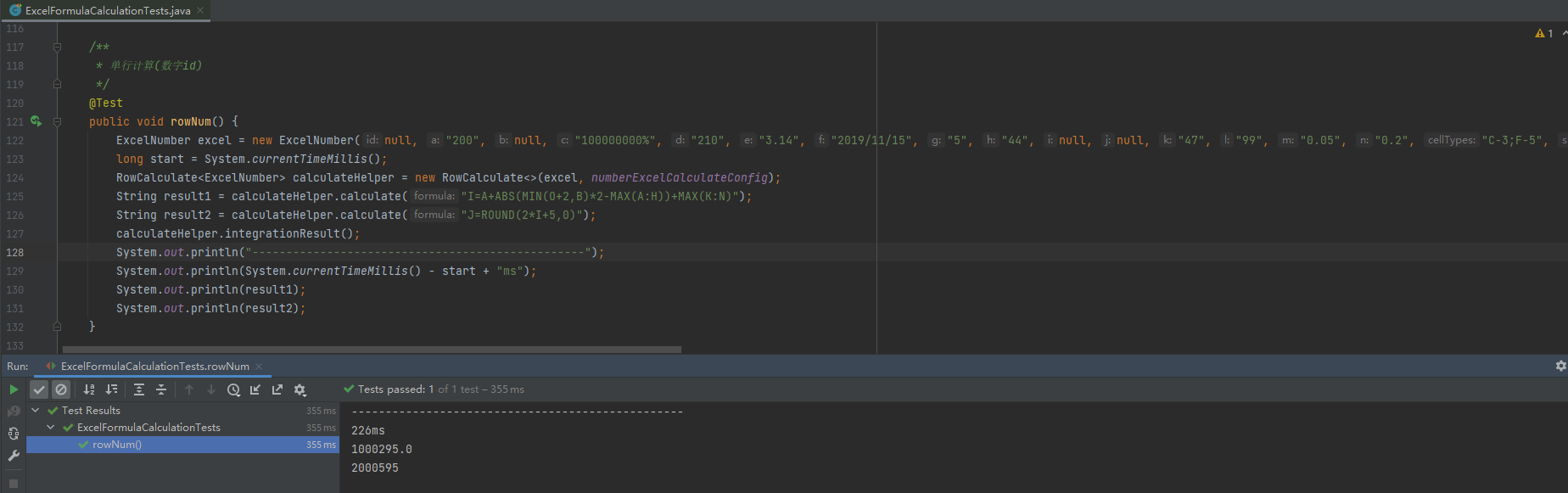自定义公式

展开全文java excel
• 的相关内容，今天继续为大家简述javaExcel公式计算和函数，并且通过实际的例子来为大家展示。首先会展示实际的代码，并附有一定的说明。一、基本计算，代码展示如下：HSSFSheetsheet=workbook.createSheet("Test...

上次已经为大家介绍过java中Excel单元格对齐包含什么？使用边框怎么做？的相关内容，今天继续为大家简述java中Excel公式的计算和函数，并且通过实际的例子来为大家展示。

首先会展示实际的代码，并附有一定的说明。

一、基本计算，代码展示如下：HSSFSheet sheet = workbook.createSheet("Test"); // 创建工作表(Sheet)

HSSFRow row = sheet.createRow(0);

HSSFCell cell = row.createCell(0);

cell.setCellFormula("2+3*4"); //设置公式

cell = row.createCell(1);

cell.setCellValue(10);

cell = row.createCell(2);

cell.setCellFormula("A 1*B 1"); //设置公式

二、SUM函数，代码展示如下：HSSFSheet sheet = workbook.createSheet("Test"); // 创建工作表(Sheet)

HSSFRow row = sheet.createRow(0);

row.createCell(0)

.setCellValue(1);

row.createCell(1)

.setCellValue(2);

row.createCell(2)

.setCellValue(3);

row.createCell(3)

.setCellValue(4);

row.createCell(4)

.setCellValue(5);

row = sheet.createRow(1);

row.createCell(0)

.setCellFormula("sum(A 1,C 1)"); //等价于"A 1+C 1"

row.createCell(1)

.setCellFormula("sum(B 1:D 1)"); //等价于"B 1+C 1+D 1"

三、日期函数，代码展示如下：HSSFSheet sheet = workbook.createSheet("Test"); // 创建工作表(Sheet)

HSSFCellStyle style = workbook.createCellStyle();

style.setDataFormat(workbook.createDataFormat()

.getFormat("yyyy-mm-dd"));

HSSFRow row = sheet.createRow(0);

Calendar date = Calendar.getInstance(); //日历对象

HSSFCell cell = row.createCell(0);

date.set(2011, 2, 7);

cell.setCellValue(date.getTime());

cell.setCellStyle(style); //第一个单元格开始时间设置完成

cell = row.createCell(1);

date.set(2014, 4, 25);

cell.setCellValue(date.getTime());

cell.setCellStyle(style); //第一个单元格结束时间设置完成

cell = row.createCell(3);

cell.setCellFormula("CONCATENATE(DATEDIF(A 1,B 1,\"y\"),\"年\")");

cell = row.createCell(4);

cell.setCellFormula("CONCATENATE(DATEDIF(A 1,B 1,\"m\"),\"月\")");

cell = row.createCell(5);

cell.setCellFormula("CONCATENATE(DATEDIF(A 1,B 1,\"d\"),\"日\")");

四、字符串相关函数，代码展示如下：HSSFSheet sheet = workbook.createSheet("Test"); // 创建工作表(Sheet)

HSSFRow row = sheet.createRow(0);

row.createCell(0)

.setCellValue("abcdefg");

row.createCell(1)

.setCellValue("aa bb cc dd ee fF GG");

row.createCell(3)

.setCellFormula("UPPER(A 1)");

row.createCell(4)

.setCellFormula("PROPER(B 1)");

接下来对上面代码中的公式作一个简要的说明：

①UPPER(string)：将文本转换成大写形式。

②PROPER(string)：将文字串的首字母及任何非字母字符之后的首字母转换成大写。将其余的字母转换成小写。

五、IF函数，代码展示如下：HSSFSheet sheet = workbook.createSheet("Test"); // 创建工作表(Sheet)

HSSFRow row = sheet.createRow(0);

row.createCell(0)

.setCellValue(12);

row.createCell(1)

.setCellValue(23);

row.createCell(3)

.setCellFormula("IF(A 1>B 1,\"A 1大于B 1\",\"A 1小于等于B 1\")");

同样地，对IF函数作一个简要的说明：IF(logical_test,value_if_true,value_if_false)用来作为逻辑判断。这里面的①Logical_test表示计算结果为TRUE或FALSE的任意值或表达式 ;

②value_if_true表示当表达式Logical_test的值为TRUE时的返回值;③value_if_false表示当表达式Logical_test的值为FALSE时的返回值。

以上就是关于java中Excel公式的计算和函数并且通过实际的例子为大家展示，同时还作了简要的说明。如果你对java知识感兴趣，想要了解更多java基础和java经典例子，敬请关注奇Q工具网。

推荐阅读：

展开全文• JAVA 自定义公式计算器， 形如EXCEL中含有大量的计算公式-财务函数： PMT FV NPER PV DB DDB 这些： 可以通过编码方式，解决在JAVA中进行数据计算， 同时可以自定义扩展自己想要的数据函数。   执行语句:...

2019独角兽企业重金招聘Python工程师标准>>>JAVA 自定义公式计算器， 形如EXCEL中含有大量的计算公式-财务函数： PMT FV NPER PV DB DDB  这些：

可以通过编码方式，解决在JAVA中进行数据计算， 同时可以自定义扩展自己想要的数据函数。

执行语句:  PMT(0.00515,360,-770000,0,0)  结果值：  4706.02254783371

执行语句:  FV(0.00515, -770000, 4706.02, 0, 1)  结果值：  913790.291262136

执行语句:  NPER(0.00515, 4706.02, -770000, 0, 1)  结果值：  360.0005643990766

执行语句:  PV(0.00515, 360, -4706.02, 0, 1)  结果值：  769999.5831231289

执行语句:  DB(100,0,10,1,12)  结果值：  100.0

执行语句:  DDB(2400,0,5,1,2)  结果值：  960.0

执行语句:  RATE(4,10000,-30000,0,0,0.1)  结果值：  0.12589832496244294

执行语句:  EFFECT(0.0525,4)  结果值：  0.05354266737075819

执行语句:  1+2*3+(3+5*6+7*(78-70))  结果值：  96

执行语句:  1+2*3+(3+5*6+(PMT(0.00515,360,-770000,0,0))*(78-70))  结果值：  37688.18038266968

执行语句:  1+2*3+(3+5*6+(PMT(RATE(4,10000,-30000,0,0,0.1),360,-770000,0,0))*(78-70))  结果值：  775573.6817686484

执行语句:  IF(30=30, IF(24=24, 0.08, 0.11), IF(24=24, 0.11, 0.14))  结果值：  0.08

执行语句:  IF(20=30,IF(24=24,0.08,0.11),IF(24=24,0.11,0.14))  结果值：  0.11

执行语句:  20.0+IF(20=30,IF(24=24,0.08,0.11),IF(24=24,0.11,0.14))  结果值：  20.11

执行语句:  (11+11+11+11+11)*(20+IF(20=30,IF(24=24,0.08,0.11),IF(24=24,0.11,0.14)))  结果值：  1106.05

执行语句:  3.0/100  结果值：  0.03

执行语句:  DEVIDE(10, 3)  结果值：  3.3333333334

执行语句:  ROUND(123.134678795, 2)  结果值：  123.13

执行语句:  ROUND(123.134678795, 0)  结果值：  123.0

执行语句:  ROUND(123.134678795, -1)  结果值：  120.0

执行语句:  (1000+1000+(1000)+(1000)+1000+1000+1000)*IF(TOINT(0.20*100)>=30,0.08,0.11)  结果值：  770.0

执行语句:  (1000+1000+(1000)+(1000)+1000+1000+1000)*IF(TOINT(0.30*100)>=30,0.08,0.11)  结果值：  560.0

执行语句:  E_NUMBER(128000*(1.2*100))  结果值：  1.536E7

执行语句:  IF(2.0<=1.6,0.05,0.10)   结果值：  0.1

执行语句:  IF(1.0<=1.6,0.05,0.10)   结果值：  0.05

执行语句:  IF(1.6<=1.6,0.05,0.10)   结果值：  0.05

执行语句:  ROUND(188888*(0.20),0)  结果值：  37778.0

执行语句:  ROUND(188888*(0.20),0)+2500+188888*IF(36=24,0.07,0.11)  结果值：  61055.68

执行语句:  ROUND(ROUND(188888*(0.20),0)+2500+188888*IF(36=24,0.07,0.11),0)   结果值：  61056.0

执行语句:  ROUNDUP(123456, -4)  结果值：  130000.0

执行语句:  NUM_DIGIT(234.0000000)  结果值：  234.0

执行语句:  NUM_DIGIT(234.0)  结果值：  234.0

执行语句:  E_YUSHU(253219, 24)  结果值：  10550.79

执行语句:  NUM_DIGIT((179999+(0)+(2500)+(24/12*(0+6700+2300)))/(24))   结果值：  8354.0

转载于:https://my.oschina.net/alexgaoyh/blog/2249004

展开全文• 7 8 import java.io.*; 9 10 public class FormulaToString { 11 12 /** 13 * @param args 14 */ 15 public void fileInput() throws IOException { 16 17 HSSFWorkbook hw = new HSSFWorkbook(new ...
• 最近接到一个需求，用Java写数据到excel，遇到一些问题，在这里请教一下大家。 　首先，我这个是在原有的excel上做修改； 　我的excel有两个sheet，在sheet2中会有引用到sheet1中单元格的求和函数； 　我写...Java POI Excel
• Java编程调用PageOffice实现从空白的excel文件动态生成excel表格，设置公式并填充数据。 集成PageOffice不但能够实现在线编辑、保存真正的Office文档，而且还可以轻松实现Word、Excel文档的动态数据填充、格式控制和...
• java导出excel自动计算公式

千次阅读 2014-08-26 09:46:27
最近在开发过程中，遇到java读取excel模板并写入数据，其中数据有计算求和的操作，一开始没有实现导出自动计算公式，所以费了一遍事，后台又重新做了一次数据计算，总感觉这样不是很好，所以上网找资料，终于在同事...excel公式
• sheet.getCellRange(currentRow,1).setValue("公式：") ; ; sheet.getCellRange(currentRow,2).setValue("结果："); //设置单元格格式 CellRange range = sheet.getCellRange(currentRow,1,currentRow,2); range....
• java是不能提供java调用excel里的函数的，所以我提供这个例子，让大家参考，必须导入jxl.jar包哦！
• 本文以通过Java代码来演示在Excel中创建及读取公式的方法。这里使用了Excel Java类库(Free Spire.XLS for Java 免费版)，在官网下载获取文件包后，解压，将lib文件夹下的jar文件导入Java程序；或者通过maven仓库下载...
• 问题：设置Excel的公式，根据java更新Excel中变量单元格，输出的Excel公式未生效 解决： //打开Excel重新计算公式 workBook.setForceFormulaRecalculation(true); 源码如下： /** * Whether the ...poi excel java
• 码农公社 210.net.cn210= 102410月24日一个...本文以通过Java代码来演示在Excel中创建及读取公式的方法。这里使用了Excel Java类库(Free Spire.XLS for Java 免费版)，在官网下载获取文件包后，解压，将lib文件夹...
• 1）EXCEL公式分类 a. 数值： 从极端情况来说，数值也是一种最简单的公式如 A1=1; b. 简单函数：简单函数指的是参数只能是数值的函数，如公式A3（简单函数）=sum(A1:A2)，,A1=1，A2=3; c. 复杂函数：复杂函数指的是...
• 针对此问题,写了一个测试,代码如下:...import java.io.FileInputStream;import java.io.FileNotFoundException;import java.io.FileOutputStream;import java.io.IOException;import org.apache.poi.hssf.user...
• 读取Excel表格，如果是 数值型 可能遇到公式 导致读取不到 switch (thecell.getCellType()) { // 读取到的是公式 case Cell.CELL_TYPE_FORMULA: String valueStr = ""; try { valueStr = String.valueOf(the...java
• 当读取Excel表格，保存至Oracle数据库时，当需要保存格式为Number时，当读取到Excel中有公式时，会报错。 使用下面的处理方式可以解决该问题。 注：代码中的参数：value就是获取后保存的值 switch (cell....Oracle poi Java Excel公式
• Java程序如何调用Excel中的各种公式针对此问,写了一个测试,代码如下:package cn.fory.formula;import java.io.FileInputStream;import java.io.FileNotFoundException;import java.io.FileOutputStream;import java....
• Java导出Excel

2021-02-27 19:41:04
前言众所周知，导Excel分为两步：抓取数据(查数据)写数据到Excel文件这两步都比较耗时间，一般我们从数据库查数据，然后组装数据，最后写数据。查数据不是本节的重点，主要是SQL，索引这一块，此处不讨论。本节重点...
• 读取excel模版的数据，然后再根据数据库里的公式进行计算例如E8+（（E7-E3）-F4-G8）/E5+（E5-H3）-S2公式有许多条，不固定格式。已经读出了excel数据，就是不知道怎么把公式里的格子替换成excel里的对应格子数据，...excel java 反射 计算
• 统计函数主要实现的是较为复杂的统计... * 主要特点：文件说明：EXCEL函数类型：统计函数 * 简单的函数如sum,average等等就不实现了 * 版本：1.0 * 制作人：刘晨曦 * 创建时间：2013-12-3 **/ package EXCEL; ...
• Java读取Excel表格公式对应的值： //伪代码org.apache.poi String fileName = file.getOriginalFilename(); //判断excel版本 if(fileName.endsWith("xls")) Workbook wb = new HSSFWorkbook(file....
• 操作excel表格用公式来处理数据时，可通过创建公式来运算数据，或通过读取公式来获取数据信息来源。这里使用了java类库(Free Spire.XLS for Java 免费版)获取文件包后，解压，将lib文件夹下的jar文件导入Java程序。...
• 7 8 import java.io.*; 9 10 public class FormulaToString { 11 12 /** 13 * @param args 14 */ 15 public void fileInput() throws IOException { 16 17 HSSFWorkbook hw = new HSSFWorkbook(new ...
• case Cell.CELL_TYPE_FORMULA: // 公式 cellValue =NumberToTextConverter.toText(cell.getNumericCellValue());//重点,无精度缺失的确切值. break; case Cell.CELL_TYPE_BLANK: // 空值 cellValue = ""; ...excel公式 poi
• 如何通过Java代码实现创建和读取Excel公式发布时间：2020-06-06 21:58:24来源：亿速云阅读：250作者：Leah如何通过Java代码实现创建和读取Excel公式？相信大部分人都还没学会这个技能，为了让大家学会，给大家总结了...
• @ApiOperation(value = "限额配置-导出Excel") @GetMapping("/exportExcel") public byte[] exportExcel(@ApiParam(value = "用户iv-user Code", required = true) @RequestHeader(value = "iv-user") String user...java 后端 oracle...

java计算excel的公式java 订阅